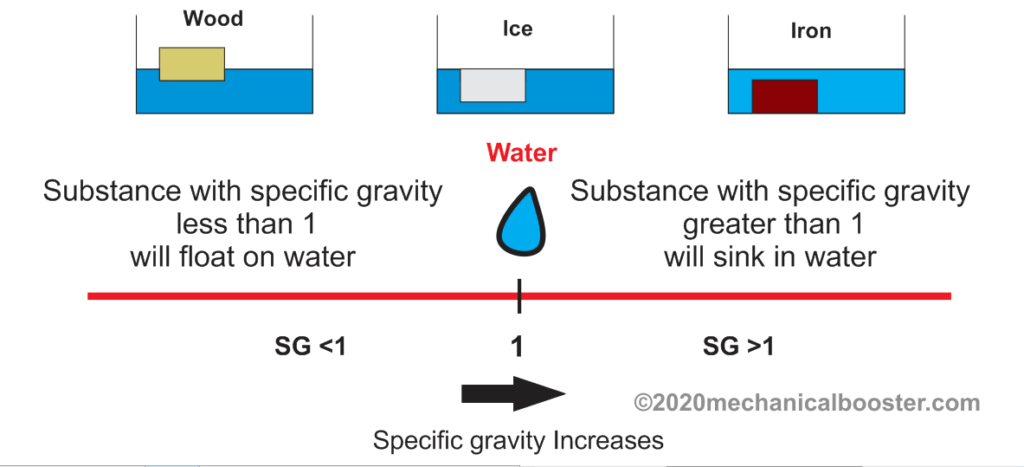# What is Specific Gravity of Water?

Three fourth of our earth is surrounded by water and without water, we cannot survive. All the living things present on the earth utilizes water for their life. But if I ask you what is the specific gravity of water and what do you mean by that then very few of you will be able to tell the answer and most of us even don’t know about what is specific gravity. So let’s begin to explore water’s specific gravity and what does it mean.

### What is specific Gravity?

It is defined as the ratio of the density of a substance to the density of a reference substance at a particular temperature and pressure.

• The reference substance is taken at a particular temperature and pressure.
• It is denoted by SG (Specific Gravity) and is a dimensionless quantity.
• It is also called as relative density.

The formula for the specific gravity is given by

Specific gravity = Density of a substance or fluid/Density of a reference substance or fluid

Here, most commonly water is taken as the reference substance. The density of water at 4 oC and 1 atm is taken as the reference for the calculation of the specific gravity of a substance.
The density of water at 4 oC and 1 atm is 1000 kg/ m3 or 1 g/cc. Now the question is that why the water at 4 oC is taken as reference. So the answer is that the water at this temperature has the highest density.

• For calculating the specific gravity of solids the density of water at 4 oC is normally taken as reference substance. And the density of water at 4 oC is 1000 kg/ m3 or 1 g/cc
• When we calculate SG for gases, the density of air at room temperature(25 oC) and at atmospheric pressure (1 atm = 101.325 kPa) is taken as reference substance. The density of air at room temperature is 1.2041 kg/ m3.

### Calculation Of Specific Gravity of Water

The Specific Gravity of water is 1.

Now the question is how this SG of Water has come. let’s explore it.

SG of water = Density of water/ Density of a reference substance

Here

density of water = 1000 kg/ m3 .

And the reference substance is water whose density is also 1000 kg/ m3

so,

SG of water = (1000 kg/ m3)/ (1000 kg/ m3)

SG of water = 1.

### What We Can Conclude From the  Specific gravitySince the specific gravity of water is 1. Now what we can conclude from this information.

• If the SG of any substance is less than one then that substance will float on water.
• If the SG of any substance is greater than one then that substance will not float on water and it will sink in water.
• And If the SG of any substance is equal to 1 then it will float just below the surface of the water.

In this article, we have learned about the specific gravity of water and how to calculate it. If you found this piece of information valuable and useful then don’t forget to like and share it.